# A risky business: how to price derivatives

December 2008

### Black-Scholes in the limit

This section contains some undergraduate level mathematics.

The Binomial model is a very simple model for understanding the ideas behind option pricing. However, so far the stock price can only take finitely many values and furthermore can only move at discrete time points. Both of these features are somewhat undesirable, and so in order to get around this we will look at the limit as the number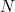of time periods tends to infinity. This will give us the celebrated Black-Scholes formula.
Firstly, we will adjust the expression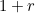for the value of the bond at time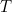to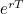. This isn't an obvious thing to do - it is itself the result of a limiting process. See Plus article Have we caught your interest for more details.

Before taking our limit, it is important that we choose our parameters so that they scale in the correct way. First of all, we fix a terminal time,, which is the expiry time of the option. Then set our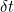to be given by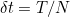. We will assume that: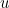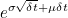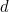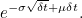Furthermore, we assume that the probability of a good or bad period is the same,that is the probability of either period is 1/2 (although as before, this probability won't matter for the option pricing). The stock price at timeis: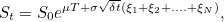(1)
where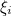is a random variable which is 1 if theth period was good and -1 otherwise. Now, we need to work out the equivalent probability,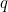, of an up jump, just as we did before. We have that: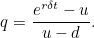If we use a Taylor expansion on the exponential terms, we see that this gives: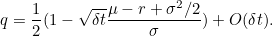We want to look at the limit astends to infinity. From our earlier analysis, we should base the price of the option on the behaviour of equation 1, but assuming that the probability of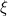being +1 is given by, rather than 1/2. The Central Limit Theorem tells us that if we have a sum of independent and identically distributed variables, which we sum and scale in the correct way, then in the limit we obtain a Normal distribution. To apply the CLT, we must first calculate the expectation of the random terms in the exponential in equation 1. Remember that we must use the probabilitiesto calculate this expectation: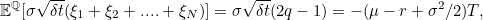where we have ignored the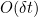terms. We have can then apply the Central Limit Theorem: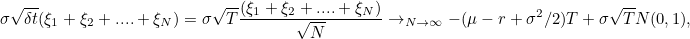where the convergence is in distribution and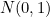denotes a Normal distribution with mean 0 and variance 1. So we see that in the limit, we should price based on the assumption that: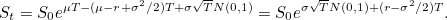Note that the true drift of the stock,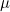, does not matter for pricing these options, since the value ofcancels in the expressions above.
We know now that we should base pricing on the formula given above. Therefore, we can now go about pricing the call option mentioned above. The price of this call option is: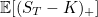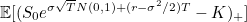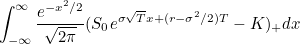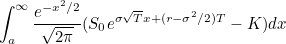where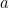is the least value of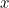such that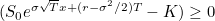, that is: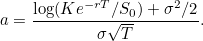We may easily compute this integral (complete the square in the exponential) to obtain the price as: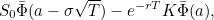where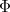is the cumulative distribution function of a standard Normal random variable and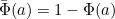. Rearrangement gives the price as: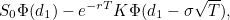where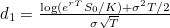. This is the famous Black-Scholes formula.Back to main article